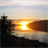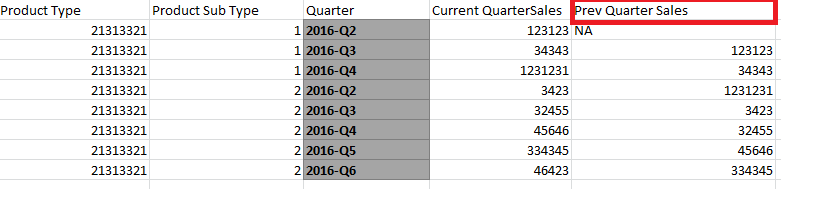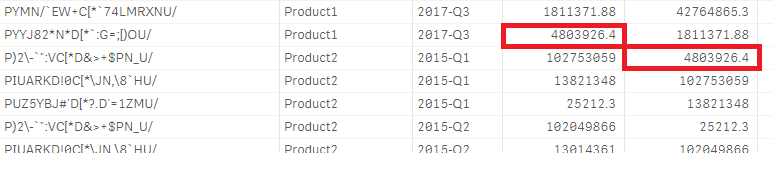# QlikView App Dev

Discussion Board for collaboration related to QlikView App Development.

cancel
Showing results for
Did you mean:Creator II

## Prev Quarter Sales Calculation in Grid table

Hi All,

We are developing a grid table where we need to create two measures

1) Current Quarter Sales

2) Previous Quarter Sales

and the grid table will contain following columnsWe are facing issues in calculating the Prev Quarter Sales measure

we have used below formulas to calculate the measure

sum({<QuarterID={"=\$((QuarterID)-1)"}>}Sales)

We have also tried using variable approach

sum({<QuarterID={\$(=\$(vPQuarterID))}>}Sales)

but we are getting zero or null values

Needed some guidance on it

I have attached sample data file  which contains sales at day level

Regards,

Bhargav

1 Solution

Accepted SolutionsMVP

Here are my questions

1) How many dimensions do you have in your chart? 2 or 3?

2) You don't want Product Type to cross over, right? Then you def. don't need TOTAL here... my first response stay... but I guess is that sorting can be off based on what you mentioned... in that case, you can give this a shot

Aggr(Above(Sum(Sales)), ProductType, YearQuarter)

Aggr function will sort first by ProductType and then by YearQuarter, no matter how the table is sorted. But the only issue that might come up is that if the sorting of YearQuarter field in the script is not done correctly, then you might see issues. These issues can be addressed if you are using QV12 or above or using Qlik Sense using The sortable Aggr function is finally here!

Aggr(Above(Sum(Sales)), ProductType, (YearQuarter, (TEXT)))

8 RepliesMVP

Before develop something, think If placed (The Right information | To the right people | At the Right time | In the Right place | With the Right context)MVP

May be you need this

Above(Sum(Sales))Creator II
Author

Hi Sunny,

We tried using this function but we get null values for rows having previous row as different product sub type

Also the data will be sorted by total sales so the previous row might contain a different quarter

Regards,

BhargavCreator II
Author

QuarterID -1 is the expression in the variableMVP

May be this

Above(TOTAL Sum(Sales))Creator II
Author

Hi Sunny,

I tried using  Above(Total sum(sales)) but there two issues

1. All the dimensions needs to be sorted properly as above function takes previous row value
2. It takes wrong value from for rows where the previous product type or sub type is different  as shown belowMVP

Here are my questions

1) How many dimensions do you have in your chart? 2 or 3?

2) You don't want Product Type to cross over, right? Then you def. don't need TOTAL here... my first response stay... but I guess is that sorting can be off based on what you mentioned... in that case, you can give this a shot

Aggr(Above(Sum(Sales)), ProductType, YearQuarter)

Aggr function will sort first by ProductType and then by YearQuarter, no matter how the table is sorted. But the only issue that might come up is that if the sorting of YearQuarter field in the script is not done correctly, then you might see issues. These issues can be addressed if you are using QV12 or above or using Qlik Sense using The sortable Aggr function is finally here!

Aggr(Above(Sum(Sales)), ProductType, (YearQuarter, (TEXT)))Creator II
Author

Hi Sunny,

Thanks a lot !!!!!

I used the following function for getting the Previous Quarter Sales

Aggr(Above(Sum([Total sales])),[Product Type],[Product Sub Type],(QuarterID,(NUMERIC, ASCENDING)))

Was not aware about the hidden features of   AGGR function.It made the calculations a lot easier

Regards,

Bhargav